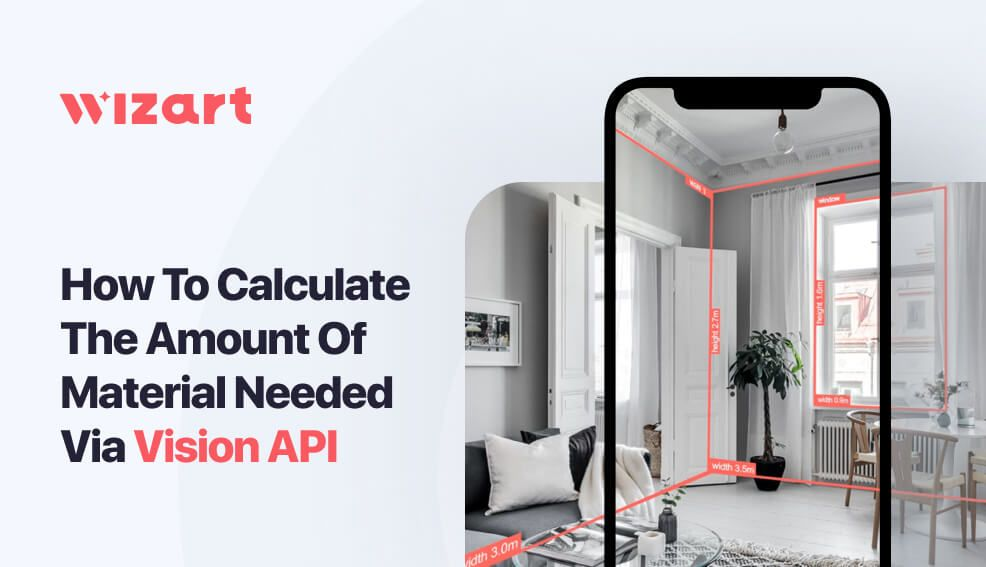# How to Calculate the Amount of Material Needed via Vision API

Technology
Artur Kuchynski
3minIf you're involved in interior design, construction, or renovation, one of the essential tasks is to calculate the surface area of the interior space, particularly the walls, floor, and ceiling.

But measuring the surface area of a room can be challenging, especially when you have to deal with complicated shapes and multiple surfaces. Fortunately, modern computer vision technology like Vision API can help you deal with it.

In this article, we'll explore how to use Vision API to calculate the visible surface area of interior spaces step-by-step. We'll be using Segmentation, Detection, and Reconstruction APIs to calculate the wall area as an example.

Interested in Vision API? Get a free trial to test its abilities →

## Step #1. Detect and segment the surfaces with Vision API

The first step is to use Segmentation API to identify and segment the target surfaces in the interior photo. The computer vision algorithms can recognize surfaces like walls, floors, and ceilings in the images and create a segmentation mask for each. You can then use these masks to calculate the visible area of the surface.

Let’s try to get the wall mask only, but first, we need to import packages and define the API Key:

```import base64
import time
from io import BytesIO

import cv2
import numpy as np
import requests
from PIL import Image

# Define API Host and your API key
API_HOST = 'https://pim-client.wizart.ai/vision-api/v3'
```

Here is the interior we will use as an example:

Now, we need to make a call to our API to get a segmentation mask:

```# Create a request body from out interior photo (we will reuse it multiple times)

# Obtain walls mask with Segmentation API
response = requests.post(f'{API_HOST}/segmentation/walls', files=body, headers={"Authorization": API_KEY})

# Decode base64 string to bytes

# Convert bytes to PIL Image object

# Convert PIL Image to NumPy array
```

And here is the result:

## Step #2. Obtain 2D surface points and surface area

Once you have the segmentation masks, you need to get the 2D surface points and surface area from the photo. Computer vision algorithms can help you identify the corners and edges of the surfaces and obtain their dimensions. For this, you need to use Detection and Reconstruction APIs:

```# Get 2D walls points with Detection API
response = requests.post(f'{API_HOST}/detection/walls', files=body, headers={"Authorization": API_KEY})
detection_data = response.json()
detection_data_wall = detection_data['walls']

# Get rid of duplacated point, the first one and the last one are always the same
detection_points = detection_data_wall['points'][:-1]

# Get the width and height of the image/mask

# Convert absolute point coordinates to pixel coordinates
point_list = [[int(point['x'] * width), int(point['y'] * height)] for point in detection_points]
```

On the other hand, the response from Reconstruction API contains an area value for each wall, so we don't need to make any additional calculations. We can simply extract the area from the response:

```# Get 3D parameters for walls with Reconstruction API
response = requests.post(f'{API_HOST}/reconstruction/walls', files=body, headers={"Authorization": API_KEY})
reconstruction_data = response.json()

# Extract wall's area
wall_reconstruction = reconstruction_data['walls']
surface_area_meters = wall_reconstruction['area']
```

## Step #3. Calculate mask area in pixels

In this step, we need to calculate the area for a particular wall, so we’ll reuse the list of points from the previous step:

```# Create empty mask for a wall polygon
# Draw the polygon on mask
# Set all pixels outside of the wall polygon to black
```

Here, the mask is the binary mask array that contains non-zeros inside the polygon and zeros outside the polygon. The np.count_nonzero function counts the number of non-zero pixels in the array, which gives us the area of the polygon in pixels.

```mask_area_px = np.count_nonzero(mask)
```

## Step 4: Calculate the visible surface area

Finally, using the segmentation masks, 2D surface points, and surface area, you can calculate the visible surface area, which should be covered with finishing materials in the interior space. You can do this using the following formula:

Visible Area (meters) = Surface Area (meters) * Mask Area (px) / Surface Area (px)

Here it’s the implementation in Python:

```def polygon_area(points):
"""Calculate the area of a polygon based on its points using the Shoelace formula."""
n = len(points)
area = 0.0
for i in range(n):
j = (i + 1) % n
area += points[i] * points[j] - points[j] * points[i]
return abs(area) / 2.0

surface_area_px = polygon_area(point_list)